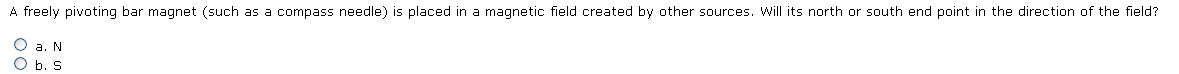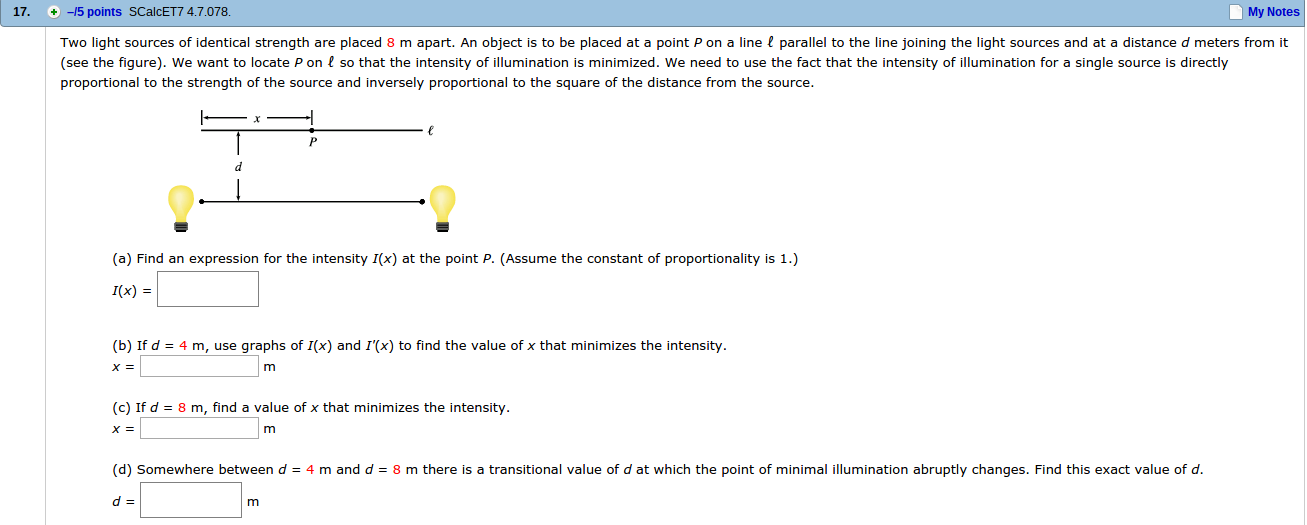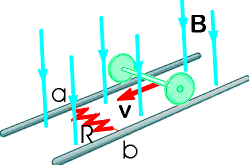Ask question

# A freely pivoting bar magnet (such as a compass needle) is placed in a magnetic field created by other sources. Will its north or south end point in the direction of the field?# A freely pivoting bar magnet (such as a compass needle) is placed in a magnetic field created by other sources. Will its north or south end point in the direction of the field?

Question
Otherasked 2021-02-17
A freely pivoting bar magnet (such as a compass needle) is placed in a magnetic field created by other sources. Will its north or south end point in the direction of the field?## Answers (1)2021-02-19
The magnetic field lines leave the north pole and enter thesouth pole. The direction of the field line is the dirction of theforce acting on a north pole. So if the field is pushing the northpole away and pulling the south pole toward it that tells you whatpole points in the direction of the north pole.
As an aside, the pole that is at the geographic north of theearth is a south magnetic pole. That is why the north pole of thecompass needle points north.

### Relevant Questionsasked 2021-04-25
A surveyor is using a magnetic compass 6.1m below apower line in which there is a steady current of 100A.
a)What is the magnetic field at the site of the compass due tothe power line?
b)Will this field interfere seriously with the compassreading?the horizontal components of Earths magnetic field at thesiter is 20uT.asked 2021-02-17
A square loop of copper wire is initially placed perpendiclar to the lines of a constant magnetic field of $$\displaystyle{5}\times{10}^{{3}}$$ T.The area enclosed by the loop is 0.2 square meter. the loopis then turned through an angle $$\displaystyle{90}^{\circ}$$ so thatthe plane of the loop is parallel to the field lines. the turntakes 0.1 second. what is the average emf induced in the loop during the turn?asked 2021-02-14
The plane of a rectangular coil, 5.0-cm by 8.0-cm, is perpendicular to the direction of a magnetic field "B". If the coil has 75 turns and a total resistance of 8.0 Ohms, at what rate must the magnitude of "B" change to induce a current of 0.10 amps in the windings of the coil?asked 2021-02-27
An alpha particle (a He nucleus, containing two protons and two neutrons and having a mass of $$\displaystyle{6.64}\cdot{10}^{{-{27}}}$$ kg) traveling horizontally at 35.6 km/s enters a uniform, vertical, 1.10 T magnetic field.
A) What is the diameter of the path followed by this alpha particle?
B) What effect does the magnetic field have on the speed of the particle?
C) What are the magnitude of the acceleration of the alpha particle while it is in the magnetic field?
D) What are the direction of the acceleration of the alpha particle while it is in the magnetic field?asked 2021-05-12
4.7 A multiprocessor with eight processors has 20attached tape drives. There is a large number of jobs submitted tothe system that each require a maximum of four tape drives tocomplete execution. Assume that each job starts running with onlythree tape drives for a long period before requiring the fourthtape drive for a short period toward the end of its operation. Alsoassume an endless supply of such jobs.
a) Assume the scheduler in the OS will not start a job unlessthere are four tape drives available. When a job is started, fourdrives are assigned immediately and are not released until the jobfinishes. What is the maximum number of jobs that can be inprogress at once? What is the maximum and minimum number of tapedrives that may be left idle as a result of this policy?
b) Suggest an alternative policy to improve tape driveutilization and at the same time avoid system deadlock. What is themaximum number of jobs that can be in progress at once? What arethe bounds on the number of idling tape drives?asked 2021-04-15
A car initially traveling eastward turns north by traveling in a circular path at uniform speed as in the figure below. The length of the arc ABC is 235 m, and the car completes the turn in 33.0 s. (Enter only the answers in the input boxes separately given.)
(a) What is the acceleration when the car is at B located at an angle of 35.0°? Express your answer in terms of the unit vectors $$\displaystyle\hat{{{i}}}$$ and $$\displaystyle\hat{{{j}}}$$.
1. (Enter in box 1) $$\displaystyle\frac{{m}}{{s}^{{2}}}\hat{{{i}}}+{\left({E}{n}{t}{e}{r}\in{b}\otimes{2}\right)}{P}{S}{K}\frac{{m}}{{s}^{{2}}}\hat{{{j}}}$$
(b) Determine the car's average speed.
3. ( Enter in box 3) m/s
(c) Determine its average acceleration during the 33.0-s interval.
4. ( Enter in box 4) $$\displaystyle\frac{{m}}{{s}^{{2}}}\hat{{{i}}}+$$
5. ( Enter in box 5) $$\displaystyle\frac{{m}}{{s}^{{2}}}\hat{{{j}}}$$asked 2021-02-10
Two light sources of identical strength are placed 8 m apart. An object is to be placed at a point P on a line ? parallel to the line joining the light sources and at a distance d meters from it (see the figure). We want to locate P on ? so that the intensity of illumination is minimized. We need to use the fact that the intensity of illumination for a single source is directly proportional to the strength of the source and inversely proportional to the square of the distance from the source.asked 2021-02-25
We will now add support for register-memory ALU operations to the classic five-stage RISC pipeline. To offset this increase in complexity, all memory addressing will be restricted to register indirect (i.e., all addresses are simply a value held in a register; no offset or displacement may be added to the register value). For example, the register-memory instruction add x4, x5, (x1) means add the contents of register x5 to the contents of the memory location with address equal to the value in register x1 and put the sum in register x4. Register-register ALU operations are unchanged. The following items apply to the integer RISC pipeline:
a. List a rearranged order of the five traditional stages of the RISC pipeline that will support register-memory operations implemented exclusively by register indirect addressing.
b. Describe what new forwarding paths are needed for the rearranged pipeline by stating the source, destination, and information transferred on each needed new path.
c. For the reordered stages of the RISC pipeline, what new data hazards are created by this addressing mode? Give an instruction sequence illustrating each new hazard.
d. List all of the ways that the RISC pipeline with register-memory ALU operations can have a different instruction count for a given program than the original RISC pipeline. Give a pair of specific instruction sequences, one for the original pipeline and one for the rearranged pipeline, to illustrate each way.
Hint for (d): Give a pair of instruction sequences where the RISC pipeline has “more” instructions than the reg-mem architecture. Also give a pair of instruction sequences where the RISC pipeline has “fewer” instructions than the reg-mem architecture.asked 2021-03-21
In the figure below, the rolling axle, 1.43 m long, is pushed along horizontal rails at a constant speed v = 3.36 m/s.A resistor R = 0.325 ohm is connected to the rails at points a and b, directly opposite each other. (The wheels make good electrical contact with the rails, and so the axle, rails, and R form a closed-loop circuit. The only significant resistance in the circuit is R.) There is a uniform magnetic field B = 0.0850 T vertically downward. Calculate the induced current I in the resistor and what horizontal force F is required to keep the axle rolling at constant speed?asked 2021-03-30
A long, straight, copper wire with a circular cross-sectional area of $$\displaystyle{2.1}{m}{m}^{{2}}$$ carries a current of 16 A. The resistivity of the material is $$\displaystyle{2.0}\times{10}^{{-{8}}}$$ Om.
a) What is the uniform electric field in the material?
b) If the current is changing at the rate of 4000 A/s, at whatrate is the electric field in the material changing?
c) What is the displacement current density in the material in part (b)?
d) If the current is changing as in part (b), what is the magnitude of the magnetic field 6.0cm from the center of the wire? Note that both the conduction current and the displacement currentshould be included in the calculation of B. Is the contribution from the displacement current significant?
...